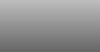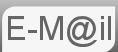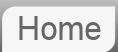# Answer to Riddle #60: 2 Egg Drop, Finding the Highest Floor.

60. There is a building of 100 floors
-If an egg drops from the Nth floor or above it will break.
-If it's dropped from any floor below, it will not break.
You're given 2 eggs.

How do you find N in the minimum number of drops?

Rumoured to be another Google or Microsoft interview. Could be, it's tough enough, plus there's plenty of scope for clues along the way. Lets crack on: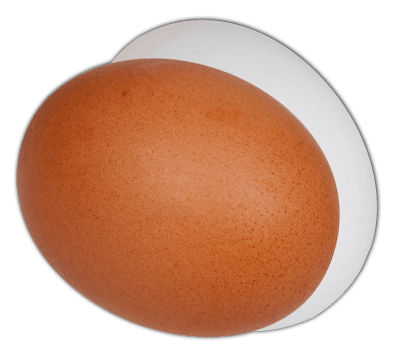Before reading the answer can I interest you in a clue?

It's worth pointing out that if an egg doesn't break it is available to use again. You can waste a lot of time befuddled if you don't get that straight away.

We're going to look at a few scenarios and see what we can learn. First we'll look at a similar problem with only one egg, then with infinite eggs, unsurprisingly our two egg solution will be a combination of the two.

## One Egg

There is nothing smart we can do here. We have to start at the bottom and work up until the egg breaks. If say we were to try and start at any floor other than 1 and the egg were to break we're stuck.

Let's say you start at 50, you might be lucky and the egg not break and you can go upwards. But if it does break there's nothing more you can do. Our only option is to start at the bottom. go up one floor at a time. The worst case scenario is that it takes 100 drops to find out. It may only take one, of course. But it could take 100.## Infinite Egg Supply

In this scenario we are trying to minimize drops with no consideration as to the number of eggs we consume. I think most people instinctively know what to do here regardless of if they formally understand the logic. It's a search and destroy, a binary search tree, high low gamble, battle ships zeroing in etc.

Let's Imagine the floor were 83.
The game is bounded by 1 and 100, so we start in the middle and try 50
The egg wont break so our new game is bound by 50 and 100. We go in the middle again, 75.
The egg still doesn't break our new game is bound 75 and 100, in the middle we guess 88.
The egg does break this time, our game is bound by 75 and 88. In the middle is 82.
The egg doesn't break. We're bound by 82 and 88. Guess 85.
The egg doesn't break. We're bound by 82 & 85. Guess 84.
The egg breaks... ...we guess 83, test it and we're there.

Tedious I suppose. But worth doing. It's probably worth asking what is the maximum number of eggs we could need for this technique. We are halving the gap each time. Starting at 100, going to 50, 25 etc. so the question is how many times can we halve 100 to end up with 1 or less. If you have the maths to solve that by logs then by all means do. But as I say if there is a non mathematical technique I'll always try and show it...

So another way of asking 'how many times can we halve 100 to end up with 1 or less' is how many times we have to double 1 to get 100 or more... And many people will know the sequence 1, 2, 4, 8, 16, 32, 64, 128, 256 etc. The maximum number of eggs is 7. Indeed with 7 eggs we can solve up to 128 (27) floors.

## Back to our two eggs

A sensible solution at this point would seem to be to combine the above perhaps use our first egg to at least narrow it to the top half or the bottom half and then use the final egg for some serious one egg solution based work. Perhaps we could employ the first egg as if it were the beginning of an infinite egg solution. Then as soon as it breaks switch to a one egg solution starting at whatever is the lowest possible value we have proven thus far. And that is a good common sense solution. But it's not optimal.

An improvement might be as follows: Lets use egg number 1 as a single egg solution on every 10th floor. The once we have determined it to the nearest ten use the second egg as a single egg solution in the 10 that we have narrowed it to. For example if the floor were 83 again we would drop the first egg at floor 10, 20, 30, 40, 50, 60, 70, 80 and then 90 where it would break. We would then use the second egg in the new range 81-90, finding floor 83 in 3 more drops.

This is a good solution. If the floor is in the 1-10 range we could discover it in 2-10 drops. However, if the floor is, say, in the 81-90 range it could take up to 18 drops. What's more if it were to be say the 19th floor we would have a spare egg as we have no reason to drop from the 20th floor twice. Remember were are trying to minimize the maximum number of drops, not the expected or average number of drops. Some thoughts around the 100th floor click here

It's clear our minimum number of drops is rising with the floor. The higher the floor the the more drops we are using with the first egg and then we have up to 9 with the second egg. It seems like if instead of going up in groups of 10 if we were to widen the gap at the bottom and decrease the gap at the top we would be able to modify the maximum number of drops so that it is consistent. That would be that the interval between first egg drops, which is the maximum number of times we could need to drop the second egg could be reduced at the same rate the number of time we have dropped the first egg.

I understand that that may not be clear at the moment. To make it clear I'm going to tell you the answer before we work it out. The table below shows the floors from which we will drop our first egg.
 Drop Floor #1 14 #2 27 #3 39 #4 50 #5 60 #6 69 #7 77 #8 84 #9 90 #10 95 #11 99 #12 100

So let's say the first egg breaks on 27 after 2 drops. We then have to narrow it from floor 15-26 with the second egg which could take from 1 to 12 drops. Making a maximum of 14.

Now consider if the first egg breaks on the 8th drop at floor 84. We must now narrow it from floor 78 - 83 which could take from 1 to 6 drops. In total a maximum of 14 drops.

So how do we arrive at that exact spacing such that the first floor is 14? We'll call the x. There is some intuition if we started at x=13 or less and tried to reduce the increment by 1 each drop we would never make it to the 100th floor, the 11th drop would be on floor 88, the 12th would be on floor 90, the 13th on 91 then were done.

If however, we started on the 15th floor, x=15, we'd be at the hundredth floor by only the 9th drop we'd be at the 99th floor which is too fast. To give equal weighting to the first and second eggs we are going to need to get to floor 100 after about x drops. This was our plan. To reduce the interval between first egg drops so that the amount of work needed to be done by the second egg decreases at the same rate. At any point the amount of work required by the second egg is x minus the number of drops performed by the first egg.

There's probably more than one way of arriving at this formula. We require the sum of all the floor increments to arrive at 100 floors or more. The sum of the increments is obviously the floor we are at. As in
 Drop Floor Increase #1 14 14 x #2 27 13 x-1 #3 39 12 x-2 #4 50 11 x-3 #5 60 10 x-4 #6 69 9 x-5 #7 77 8 x-6 #8 84 7 x-7 #9 90 6 x-8 #10 95 5 x-9 #11 99 4 x-10 #12 100

We require the sum of the increments to be 100 or greater. We are summing the values x, x-1, x-2, x-3... ...1 and require that this be greater than or equal to 100. There is a formula for the sum of the first x natural numbers. (For a short explanation of the formula click here.)

Which looks like this (x+1)x/2

(x+1)x/2 ≥ 100
x ≥ 13.650971698
as the positive root.

Which we round for the value of x the first time the sum of x natural numbers if over 100 to 14.

## Further Work

There's clearly a lot further we could go with this. We now have a formula for any number of floors with 2 eggs, for 1000 floor trivially we can show you start on the 45th floor, using the same technique. But what about the number of eggs....
The problem is recursive. If you had say 3 eggs, once it breaks you then have a two egg problem in the window left by the first. I'm going to leave it here for now. But we'll see.

## Assumptions

There is a chance that an egg dropped from the 90th floor might not break? Seriously? Obvious nonsense. Other than that... That it's clearly defined what we mean by a break, there is no cumulative damage.

Bard completely got this one wrong. Perhaps because it had heard the problem before ChatGPT got it right.

If you're curious what Bard made of this puzzle...

If you're curious what ChatGPT made of this puzzle...

© Nigel Coldwell 2004 -  – The questions on this site may be reproduced without further permission, I do not claim copyright over them. The answers are mine and may not be reproduced without my expressed prior consent. Please inquire using the link at the top of the page. Secure version of this page.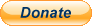PayPal
I always think it's arrogant to add a donate button, but it has been requested. If I help you get a job though, you could buy me a pint! - nigel